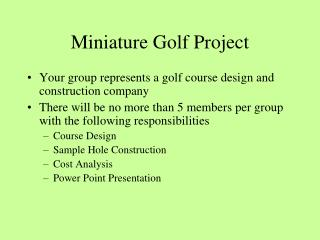DownloadDownload PresentationMiniature Golf Project

# Miniature Golf Project

Download Presentation## Miniature Golf Project

- - - - - - - - - - - - - - - - - - - - - - - - - - - E N D - - - - - - - - - - - - - - - - - - - - - - - - - - -
##### Presentation Transcript

1. Miniature Golf Project • Your group represents a golf course design and construction company • There will be no more than 5 members per group with the following responsibilities • Course Design • Sample Hole Construction • Cost Analysis • Power Point Presentation

2. Course Design • Make a scale drawing of your 9 hole golf course • Find area, perimeter, and direct distance to hole • Be sure not to count interior edges between different areas • Use color • “Pocket” diameter is 3in., but you can use a point in the sketch • Show the scale for each hole • Assume all holes are flat

3. Individual Hole Design Requirements • Design your hole using geometric shapes • Calculate the area, perimeter • Show hole-in-one route with a dotted line • You should always make it possible to get a hole-in-one • Adjust hole shape if necessary • Calculate the distance required for a hole-in-one • Decide on realistic par for each hole (at least 2)

4. Sample Hole 1 • Area (with “pocket”) = 32.27 sq.ft • Perimeter = 35.91ft • Distance = 10.58ft • Par 2

5. Sample Hole 2 • Area (with “pocket”) = 33.62 sq.ft • Perimeter = 43.89ft • Distance = 8.95ft • Par 2

6. Sample Hole 3 • Area (w/“pocket”, w/o bumpers) = 21.35 sq.ft • Perimeter (w/bumper edges) = 40.59ft • Distance = 8.78ft • Par 3

7. Sample course layout • Uses sample holes 2,3 • Use rotations and dilations

8. Portfolio • Portfolio (13 page minimum) • Cover page—school appropriate company name and logo • One page for each sample hole • One course layout diagram • An accounting sheet with cost breakdown • A sample score card listing par and direct length for each hole

9. Model Hole • Make a model of one hole on your course • Use cardboard, wood, styrofoam, whatever works best • This model must be life – size as we will be using it to play golf • Calculate area and perimeter using geometric formulae

10. Marketing • Decide on a school appropriate company name, a golf course name, and golf course theme • Design the score card, listing par and direct length for each hole

11. Accounting • You will need to come up with the costs of construction using the following data (and ignoring labor, etc.) • Turf:\$1.25/sq.ft • Trim around hole,bumpers: \$0.25/ft. • Cement bumpers:\$2.50/sq.ft • “Pocket”:\$5 each • Calculate the following: • Costs for each hole • Cost for each material • Total costs

12. Technical Support • Put all documents from your group members into a PowerPoint presentation • Make it look good! • Explain all the geometry used in the design of the course

13. Assessment • Each group is responsible for a • PowerPoint presentation • Portfolio consisting of a • Cover page—school appropriate company name and logo • One page for each sample hole • One course layout diagram • An accounting sheet with cost breakdown • A sample score card • Model of a hole • Every group member gets the same grade, except for obvious exceptions • This project is worth a test grade. • Your project is due May 9!! • GET ORGANIZED ASAP

14. Assessment Rubric